# User:IssaRice/Linear algebra/Invertible equals expressible as change of coordinate matrix

Let$T : \mathbf F^n \to \mathbf F^n$ be invertible. We want to write$T$ as a change of coordinate matrix, i.e. as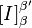$[I]_\beta^{\beta'}$ for some appropriate choice of bases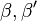$\beta,\beta'$. More precisely we want to write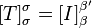$[T]_\sigma^\sigma = [I]_\beta^{\beta'}$ where$\sigma = (e_1, \ldots, e_n)$ is the standard basis of$\mathbf F^n$. If we pick$\beta := (Te_1, \ldots, Te_n)$ and$\beta' := \sigma$, then the kth column of$[I]_\beta^{\beta'}$ is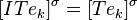$[ITe_k]^\sigma = [Te_k]^\sigma$, which is the kth column of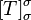$[T]_\sigma^\sigma$. Where did we use invertibility of$T$? To show that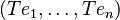$(Te_1, \ldots, Te_n)$ is a basis requires this; see User:IssaRice/Linear algebra/Properties of a list of vectors and their images.
The matrix$[I]_\beta^{\beta'}$ is invertible and its inverse is$[I]_{\beta'}^\beta$.
More generally, let$V$ be a finite-dimensional vector space and let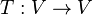$T:V\to V$ be an invertible linear transformation. Can we write$T$ as a change of coordinate matrix? One problem is that the question doesn't quite make sense. If our vectors aren't in$\mathbf F^n$, then what does it mean to multiply a matrix by a vector? In the case of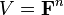$V = \mathbf F^n$ we could use the fact that$v = [v]^\sigma$.
This suggests that we can just pick a basis, say$\alpha$, and use it as our "standard" basis. Now we have$[T]_\alpha^\alpha = [I]_{(Tv_1, \ldots, Tv_n)}^\alpha$.
Recall that there is a unique linear map that sends a basis to some list of vectors, and that the list of image vectors is a basis iff(?) the map is invertible. How can we interpret the result of this page in this context? If we start out with the standard basis$(e_1, \ldots, e_n)$ then$T$ sends this to the basis$(Te_1, \ldots, Te_n)$. But now the result says if we start with the basis$(Te_1, \ldots, Te_n)$ and, without doing anything, just change our perspective to look at these vectors in terms of$(e_1, \ldots, e_n)$, then this "perspective change operation" is identical to applying$T$. This sounds crazy, and I can't visualize it!